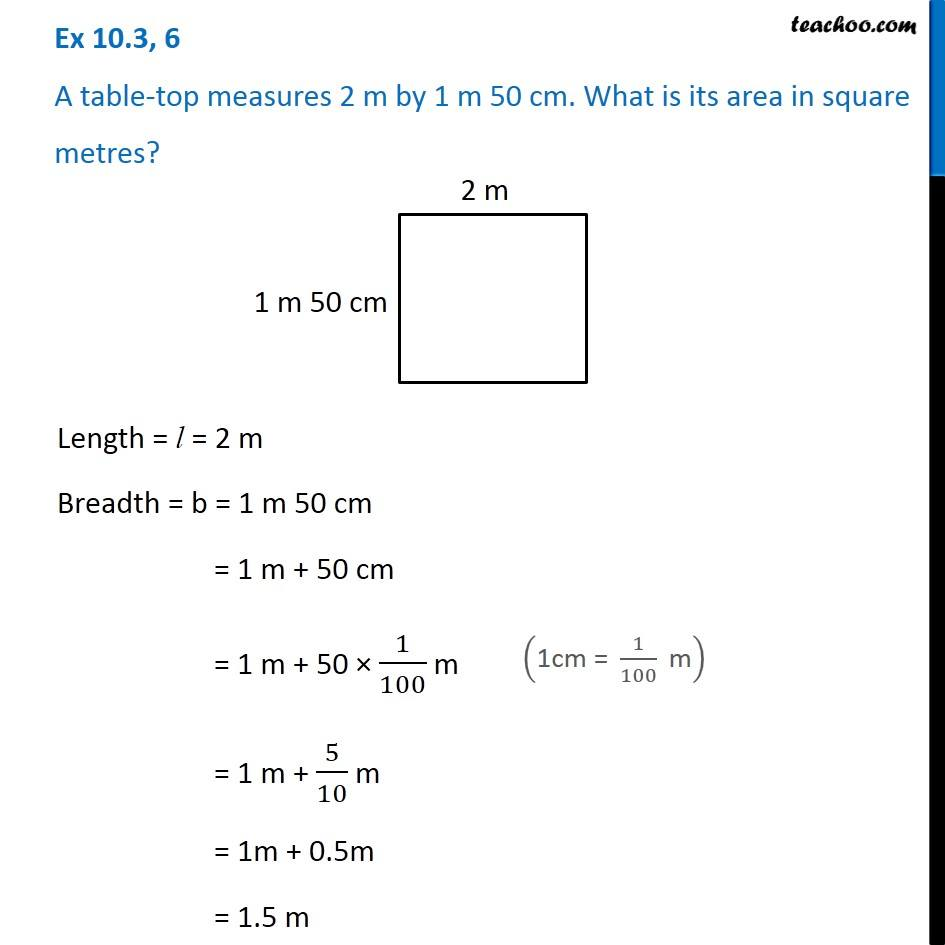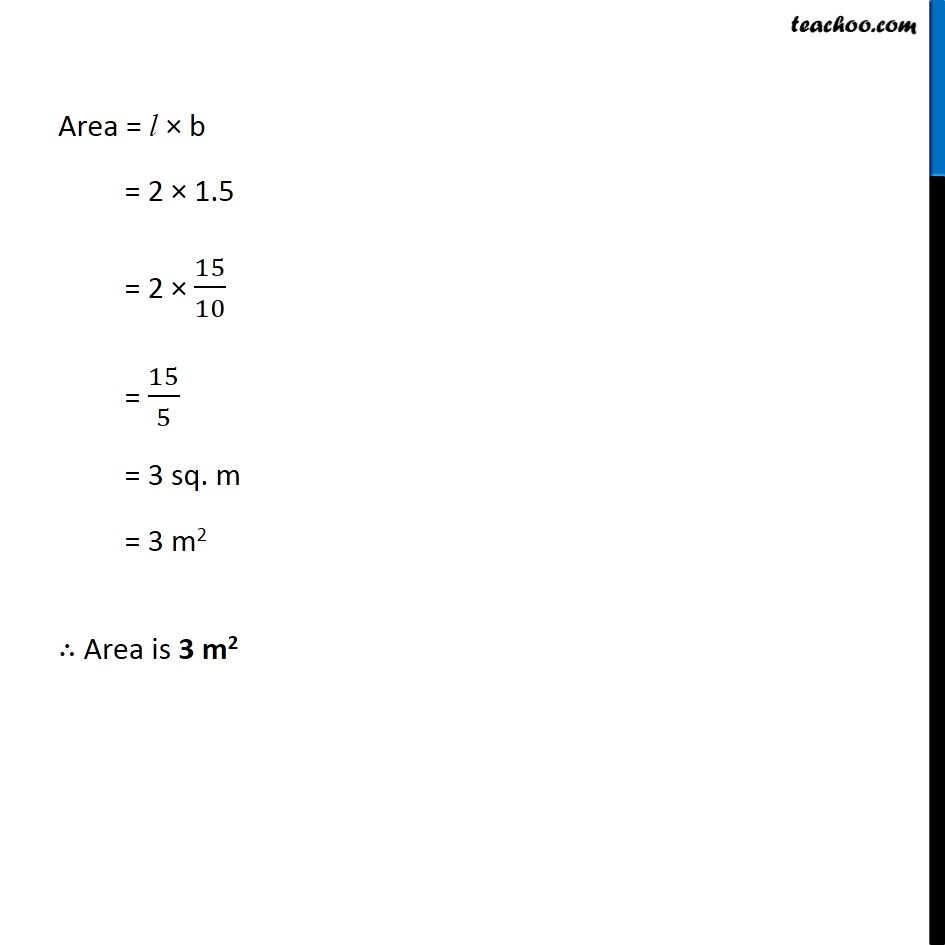Subscribe to our Youtube Channel - https://you.tube/teachoo

1. Chapter 10 Class 6 Mensuration
2. Concept wise
3. Finding Area by Changing units

Transcript

Ex 10.3, 6 A table-top measures 2 m by 1 m 50 cm. What is its area in square metres? Length = l = 2 m Breadth = b = 1 m 50 cm = 1 m + 50 cm = 1 m + 50 × 1/100 m = 1 m + 5/10 m = 1m + 0.5m = 1.5 m ("1cm = " 1/100 " m" ) Area = l × b = 2 × 1.5 = 2 × 15/10 = 15/5 = 3 sq. m = 3 m2 ∴ Area is 3 m2

Finding Area by Changing units